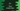# C++ program to addition, subtraction, multiplication and division using a class## C++ program to addition, subtraction, multiplication and division using a class:

In this post, we will learn how to create a class in C++ and how to do different operations like addition, subtraction, multiplication and division using that class object.

### How to find the addition, subtraction, multiplication and division of two numbers:

We have to use +, -, * and / operators.

• + is used for addition. For example 1 + 2 is 3
• - is used for subtraction. For example 10 - 5 is 5
• * is used for multiplication. For example 5 * 4 is 20
• / is used for division. For example 10/2 is 5.

Before we solve it using a class, let me show you how it works if we don’t use a class.

### C++ program to find the addition, subtraction, multiplication and division of two numbers:

Let’s take a look at the below program:

``````#include <iostream>
using namespace std;

int main()
{
double firstNumber, secondNumber;

cout << "Enter the first number: " << endl;
cin >> firstNumber;

cout << "Enter the second number: " << endl;
cin >> secondNumber;

cout << "firstNumber + secondNumber = " << firstNumber + secondNumber << endl;

cout << "firstNumber - secondNumber = " << firstNumber - secondNumber << endl;

cout << "firstNumber * secondNumber = " << firstNumber * secondNumber << endl;

cout << "firstNumber/secondNumber = " << firstNumber / secondNumber << endl;
}``````

Here,

• firstNumber and secondNumber are two double variables to hold the first and the second numbers.
• It is reading the numbers as inputs from the user. cout is used to print a message on the console and cin is used to read and assign the value.
• The last 4 cout are used to print the addition, subtraction, multiplication and division of the numbers user has entered.

If you run this program, it will print output as like below:

``````Enter the first number:
120
Enter the second number:
40
firstNumber + secondNumber = 160
firstNumber - secondNumber = 80
firstNumber * secondNumber = 4800
firstNumber/secondNumber = 3

Enter the first number:
124.56
Enter the second number:
122.2
firstNumber + secondNumber = 246.76
firstNumber - secondNumber = 2.36
firstNumber * secondNumber = 15221.2
firstNumber/secondNumber = 1.01931``````

### C++ program to find the addition, subtraction, multiplication and division of two numbers using a class:

Let’s use a class and re-write the above program:

``````#include <iostream>
using namespace std;

class Calculator
{
public:
void printResult(double x, double y)
{
cout << "firstNumber + secondNumber = " << x + y << endl;

cout << "firstNumber - secondNumber = " << x - y << endl;

cout << "firstNumber * secondNumber = " << x * y << endl;

cout << "firstNumber/secondNumber = " << x / y << endl;
}
};

int main()
{
double firstNumber, secondNumber;
Calculator c;

cout << "Enter the first number: " << endl;
cin >> firstNumber;

cout << "Enter the second number: " << endl;
cin >> secondNumber;

c.printResult(firstNumber, secondNumber);
}``````

In this program,

• We created a new class Calculator. This class has one public method called printResult. This method takes two double numbers and prints the addition, subtraction, multiplication and division of these numbers.
• In the main method, we are taking the numbers as inputs from the user and storing them in firstNumber and secondNumber variables.
• It created one object of Calculator and calls the printResult method to print the result.

If you run this program, it will give similar output as the above program:

``````Enter the first number:
10
Enter the second number:
20
firstNumber + secondNumber = 30
firstNumber - secondNumber = -10
firstNumber * secondNumber = 200
firstNumber/secondNumber = 0.5``````

### Using a class and separate method for addition, subtraction, division and multiplication:

The above example uses a class but we can also divide the tasks into different methods. i.e. we can create separate methods to calculate the addition, subtraction, division and multiplication. Each method will take two numbers as the parameters and return the result.

``````#include <iostream>
using namespace std;

class Calculator
{
public:
{
return x + y;
}

double subtract(double x, double y)
{
return x - y;
}

double multiply(double x, double y)
{
return x * y;
}

double divide(double x, double y)
{
return x / y;
}
};

int main()
{
double firstNumber, secondNumber;
Calculator c;

cout << "Enter the first number: " << endl;
cin >> firstNumber;

cout << "Enter the second number: " << endl;
cin >> secondNumber;

cout << "firstNumber + secondNumber = " << c.add(firstNumber, secondNumber) << endl;

cout << "firstNumber - secondNumber = " << c.subtract(firstNumber, secondNumber) << endl;

cout << "firstNumber * secondNumber = " << c.multiply(firstNumber, secondNumber) << endl;

cout << "firstNumber/secondNumber = " << c.divide(firstNumber, secondNumber) << endl;
}``````

Here, we added four new public methods to the Calculator class. We are calling these methods from the Calculator object in the main method. If you run this program, it will print similar output.

But it has advantages over the above examples. Calculator class is responsible only to calculate the results. It is not responsible to anything like printing the message. So, we can use this class from different classes and use different messages with the results.

``````Enter the first number:
100
Enter the second number:
123
firstNumber + secondNumber = 223
firstNumber - secondNumber = -23
firstNumber * secondNumber = 12300
firstNumber/secondNumber = 0.813008``````